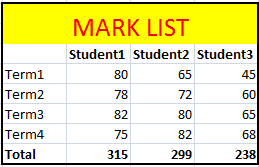# Format Excel Page in VB.NET

When working with Excel in VB.Net, you have the ability to interact with the various objects provided by the Excel object model. This allows you to perform tasks such as creating worksheets, reading data from worksheets, and formatting cells, among others, directly from your VB.Net application. In this article, we will explore the process of manipulating Excel cells in more detail within the context of a VB.Net application.

The following source code utilizes the Microsoft Excel 12.0 Object Library. In the previous section, we learned how to import the Microsoft Excel 12.0 Object Library into a VB.Net project. In order to access the object model from Visual VB.Net, it is necessary to add the Microsoft Excel 12.0 Object Library to your project. For a step-by-step guide on how to add the Excel library to your project, please refer to the previous chapter.

## Format Excel Column (or Cell)

### Format Excel cells to store values as text

Formating Excel cells to text format will solve the problem of losing leading zeo values when you import data from other data sources to excel using VB.Net.

Dim formatRange As Excel.Range formatRange = xlWorkSheet.Range("a1", "b1") formatRange.NumberFormat = "@" xlWorkSheet.Cells(1, 1) = "098"

### Excel Number Formatting

Dim formatRange As Excel.Range formatRange = xlWorkSheet.Range("a1", "b1") formatRange.NumberFormat = "#,###,###" xlWorkSheet.Cells(1, 1) = "1234567890"

### Excel Currency Formatting

Dim formatRange As Excel.Range formatRange = xlWorkSheet.Range("a1", "b1") formatRange.NumberFormat = "\$ #,###,###.00" xlWorkSheet.Cells(1, 1) = "1234567890"

### Excel Date Formatting

Dim formatRange As Excel.Range formatRange = xlWorkSheet.Range("a1", "b1") formatRange.NumberFormat = "mm/dd/yyyy" 'formatRange.NumberFormat = "mm/dd/yyyy hh:mm:ss"; xlWorkSheet.Cells(1, 1) = "31/5/2014"

## Bold the fonts of a specific row or cell

### Bold entire row of an Excel Document

Dim formatRange As Excel.Range formatRange = xlWorkSheet.Range("a1") formatRange.EntireRow.Font.Bold = True xlWorkSheet.Cells(1, 5) = "Bold"

### Bold specific cell

workSheet.Cells(2, 1).Font.Bold = True

## Add border to a specific cell

Dim formatRange As Excel.Range = xlWorkSheet.UsedRange Dim cell As Excel.Range = formatRange.Cells(3, 3) Dim border As Excel.Borders = cell.Borders border.LineStyle = Excel.XlLineStyle.xlContinuous border.Weight = 2.0

## Border around multiple cells in excel

Dim formatRange As Excel.Range formatRange = xlWorkSheet.Range("b2", "e9") formatRange.BorderAround(Excel.XlLineStyle.xlContinuous, Excel.XlBorderWeight.xlMedium, Excel.XlColorIndex.xlColorIndexAutomatic, Excel.XlColorIndex.xlColorIndexAutomatic)

## Excel Cell coloring

### Cell background color

Dim formatRange As Excel.Range formatRange = xlWorkSheet.Range("b1", "b1") formatRange.Interior.Color = System.Drawing.ColorTranslator.ToOle(System.Drawing.Color.Red) xlWorkSheet.Cells(1, 2) = "Red"

## Cell font color , size

Dim formatRange As Excel.Range formatRange = xlWorkSheet.Range("b1", "b1") formatRange.Font.Color = System.Drawing.ColorTranslator.ToOle(System.Drawing.Color.Red) formatRange.Font.Size = 10 xlWorkSheet.Cells(1, 2) = "Red"

## Excel Styles to named range

Dim myStyle As Excel.Style = Globals.ThisWorkbook.Styles.Add("myStyle") myStyle.Font.Name = "Verdana" myStyle.Font.Size = 12 myStyle.Font.Color = System.Drawing.ColorTranslator.ToOle(System.Drawing.Color.Red) myStyle.Interior.Color = System.Drawing.ColorTranslator.ToOle(System.Drawing.Color.Gray) myStyle.Interior.Pattern = Excel.XlPattern.xlPatternSolid

## How to merge Excel cells

chartRange = xlWorkSheet.Range("b2", "e3") chartRange.Merge()